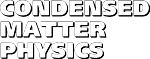Condensed Matter Physics, 2013, vol. 16, No. 1, 13002
DOI:10.5488/CMP.16.13002           arXiv:1303.5211

Title: How to solve Fokker-Planck equation treating mixed eigenvalue spectrum?
Author(s):
 M. Brics ( Institute of Physics, Rostock University, D-18051 Rostock, Germany ) , J. Kaupuzs ( Institute of Mathematics and Computer Science, University of Latvia, LV-1459 Riga, Latvia ) , R. Mahnke ( Institute of Physics, Rostock University, D-18051 Rostock, Germany )

An analogy of the Fokker-Planck equation (FPE) with the Schrödinger equation allows us to use quantum mechanics technique to find the analytical solution of the FPE in a number of cases. However, previous studies have been limited to the Schrodinger potential with discrete eigenvalue spectrum. Here we will show how this approach can be applied also for mixed eigenvalue spectrum with bounded and free states. We solve the FPE with boundaries located at x=±L/2 and take the limit L→∞, considering examples with constant Schrödinger potential and with Pöschl-Teller potential. An oversimplified approach has been earlier proposed by M.T. Araujo and E. Drigo Filho. A detailed investigation of the two examples shows that the correct solution, obtained in this paper, is consistent with the expected Fokker-Planck dynamics.

Key words: Fokker-Planck equation, Schrödinger equation, Pöschl-Teller potential
PACS: 05.10.Gg

 Full text [pdf, ps] << List of papers